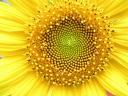#Drama! Intrigue! Geekiness!

## September 12, 2007

### The calculative artist..

ConnectCracked by: srikanth , abhinav , Vinod , udupendra , Bikiran , bs , Mahesh Herle , nihit , Arun Krishnamoorthy , piezocake , Gammafunction , Sharath , Preetish , Sandesh , alephnull , Non Descript , yaksha , bobo , Abhishek.

Prasad gets a deeper connect. I wonder what he was on :P

Fibonacci Sequence..

1. Sunflower Florets form spirals in 34 and 55, which is a Fibonacci Sequence. Also, like Mahesh Herle points out, the number of petals in any flower is a fibonacci number

2. xkcd’s fibonacci reference.. the dude starts counting the number of times his gf touches him and associates it to the fibonacci sequence.

### 22 Responses to “The calculative artist..”

1. srikanth
 The fibonacci sequence. 1) A number in this sequence is supposedly an efficient number of petals for a flower to have. Ref: http://www.popmath.org.uk/rpam.....lower.html 2)http://xkcd.com/289/ . ’nuff said.

2.  This is the Fibonacci Series Too much of under the sheets touching ! hehe .

3. Vinod
 The connect is the Fibonacci Sequence. 1.The shaded portion in the comic strip “Alone” is that, 2.The Sunflower’s florets display the arrangement in concordance with the Fibonacci numbers.

4. udupendraFibonacci series. Sunflower florets – 34, 55, 89 Making out the Fibonacci way in xkcd

5. Bikithe golden ratio ….

6. Bikialternately could be the Fibonacci series …

7. Bikisorry for the multiple posting … :) the connect is fibonacci series / golden ratio ….

8. bs
 Fibonacci series – the spirals on the sunflower follow the series. And in typical xkcd fixation with elementary math-pseudogeekery, the ‘touch-touch’ counts are 1,2,3,5

9. Mahesh Herle
 The masked text is “Fibonacci numbers”. And the connection of Fibonacci numbers with flowers is that, the number of petals of Sunflower ( i think its _any_ flower ) is generally a fibonacci number.

10. nihitThe Fibonacci Sequence…

11.  Does this have anything to do with Fibonacci or the Divin Proportion/Golden Ratio

12. piezocake
 Fibonacci series.

13. GammafunctionThe Fibonacci series.1st pic:Sunflower head displaying florets in spirals of 34 and 55 around the outside constructed by ‘nature’ using the sequence.

14. Sharath
 Its Fibonacci!!!! Leonardo Fibonacci. You see the Fibonacci numbers in the spirals of the sunflowers (“girasole” in Italian).

15. Preetish
 The Fibonacci sequence is the connect ..

16. Sandesh
 Fibonacci series………

17. alephnull
 Fibonacci sequence. The two interleaved spirals of seeds in a sunflower are consecutive Fibonacci numbers.

 The Golden Ratio? The model for the pattern of florets in the head of a sunflower is distantly related to the Golden Ration (Polar co-ordinates for the florets around the center use the golden ration). The ratio between the part of him that he feels closest to whatever he’s supposed to be feeling close to and the part which is feeling distant follows the golden ratio. The ratio of the hands and legs of the stick figures are in the golden ratio. The ratio of the sides of the rectangular pillow the dude is resting his head on also follows the golden ratio. As does the bed spread and the bed.

19. Non Descript
 The Fibonacci sequence. Florets in the sunflower are arranged according to this sequence

20. yakshaThe calculative artist is Fibonacci The connect explained in detail here: http://britton.disted.camosun......bslide.htm

21.  Fibonacci sequence. Sunflowers – opposing spirals of seeds due to the F sequence. Cartoon from xkcd, fibonacci blanked out.

22. AbhishekFibonacci numbers

« Previous « [static] « | » Look at it this way: in a hundred years, who’s gonna care? » Next »